# Hash Tables

## Arrays

Balanced search tree and binary heap data structures guarantee that the height of the tree will be roughly logarithmic with respect to its total size, resulting in tree operations such as search and insertion only taking logarithmic time. This is a massive improvement over linear search. A tree containing over 1 million elements has a height that’s within a constant factor of 20!

How can we do even better than a balanced search tree? Java’s `TreeSet` and `TreeMap` classes are represented as red-black trees. In this lesson, we’ll examine hash tables, the data structure behind Java `HashSet` and `HashMap`, which can have constant time search and insertion—regardless of whether your hash table contains 1 million, 1 billion, 1 trillion, or even more elements.

Contiguous memory arrays
Arrays are stored contiguously in memory where each array index is next to each other, one after the other. Unlike a linked list, arrays do not need to follow references randomly throughout memory. Indexing into an array is a constant-time operation as a result.

The `DataIndexedIntegerSet` uses this idea of an array to implement a set of integers. It maintains a `boolean[] present` of length 2 billion, one index for almost every non-negative integer. The boolean value stored at each index `present[x]` represents whether or not the integer `x` is in the set.

`void add(int x)`
Adds `x` to the set by assigning `present[x] = true`.
`boolean contains(int x)`
Returns the value of `present[x]`.
`void remove(int x)`
Removes `x` from the set by assigning `present[x] = false`.
``````public class DataIndexedIntegerSet {
private boolean[] present;

public DataIndexedIntegerSet() {
present = new boolean;
}

present[x] = true;
}

public boolean contains(int x) {
return present[x];
}

public void remove(int x) {
present[x] = false;
}
}
``````

While this implementation is simple and fast, it takes a ton of memory to store the 2-billion length `present` array—and it doesn’t even work with negative integers! Additionally, we’ll also need to come up with a way to generalize this idea so that it works with other data types such as strings.

Let’s focus on the second problem. Say we want to add “cat” to an array-based set of strings. One way to determine where to store “cat” is to use the first character ‘c’ to determine the array index. We can store words with first character ‘a’ at index 1, ‘b’ at index 2, ‘c’ at index 3, etc.

Give an example where this idea doesn't work as expected.

There are many words that start with the character ‘c’! After adding “cat” to the set, `contains("chupacabra")` will also return true even though the client never called `add("chupacabra")`.

## Collisions

A collision occurs when two or more keys share the same index. One way we can avoid collisions is to be more careful about how we assign each English word. If we make sure every English word has its own unique integer index, then we won’t run into collisions!

Suppose the string “a” goes to 1, “b” goes to 2, “c” goes to 3, …, and “z” goes to 26. Then the string “aa” should go to 27 since (1 ⋅ 261) + (1 ⋅ 260) = 27, “ab” to 28, “ac” to 29, and so forth. Since there are 26 lowercase English characters, our base is 26.

Generalizing this pattern, we can compute the index for “cat” as (3 ⋅ 262) + (1 ⋅ 261) + (20 ⋅ 260) = 2074. This mathematical formula is known as a hash function. The result of applying this hash function to the string “cat” is the hash value 2074.

Hash function
Returns an integer representation of a given object called its hash code.

So long as we pick a base that’s at least 26, this hash function is guaranteed to assign each lowercase English word a unique integer. But it doesn’t work with uppercase characters or punctuation. If we want to support other languages than English, we’ll need an even larger base. For example, there are 40,959 characters in the Chinese language alone. A `char` in Java supports all of these characters and more: to support all strings, the base would need to be 216.

To make matters worse, the maximum size of an array in Java is limited to the size of the largest Java `int`, which is 2,147,483,647. There are more unique strings than there are unique Java `int` values, so collisions are inevitable!

### Question 1

Compute the hash code for “bee” using the hash function above.

Explanation

Since the character ‘b’ is the second letter in the alphabet and ‘e’ is the fifth, we get (2 ⋅ 262) + (5 ⋅ 261) + (5 ⋅ 260) = 1487.

## Separate chaining

Hash tables improve upon data-indexed arrays by handling collisions. We’ll primarily study separate chaining hash tables, though there are other ways of handling collisions.

Separate chaining
A strategy for handling collisions that replaces the `boolean[] present` with an array of “buckets” where each bucket starts empty. For adding an item `x` that belongs at index `h`, add `x` to bucket `h`. To avoid duplicates (as required by the set and map ADTs), only add `x` if it is not already present in the bucket.

Since separate chaining addresses collisions, we can now use smaller arrays. Instead of directly using the hash code as the index into the array, take the modulus of the hash code to compute an index into the array.

Separate chaining comes with a cost. The worst-case runtime for adding, removing, or finding an item is now in Θ(Q) where Q is the size of the largest bucket. Consider how we might implement `contains`: rather than just returning the true/false boolean value, we’ll need to search through the bucket. The runtime of a separate chaining hash table is affected by the bucket data structure.

`contains(Object o)`
Compute the hash code of `o` modulo the length of the array to get the bucket index. Then, search the bucket by calling `equals` on each item.## Hash table insertion

Consider the following code snippet.

``````Map<Integer, String> map = new HashMap<>();
map.put(20,  "green");
map.put(2,   "red");
map.put(10,  "blue");
map.put(4,   "green");
map.put(105, "green");
map.put(102, "yellow");
map.put(38,  "purple");
``````

### Question 1

Assuming the `HashMap` does not resize and its underlying array is fixed at 5 buckets, which of the following diagrams might represent the state of the hash table at the end of the code snippet?

•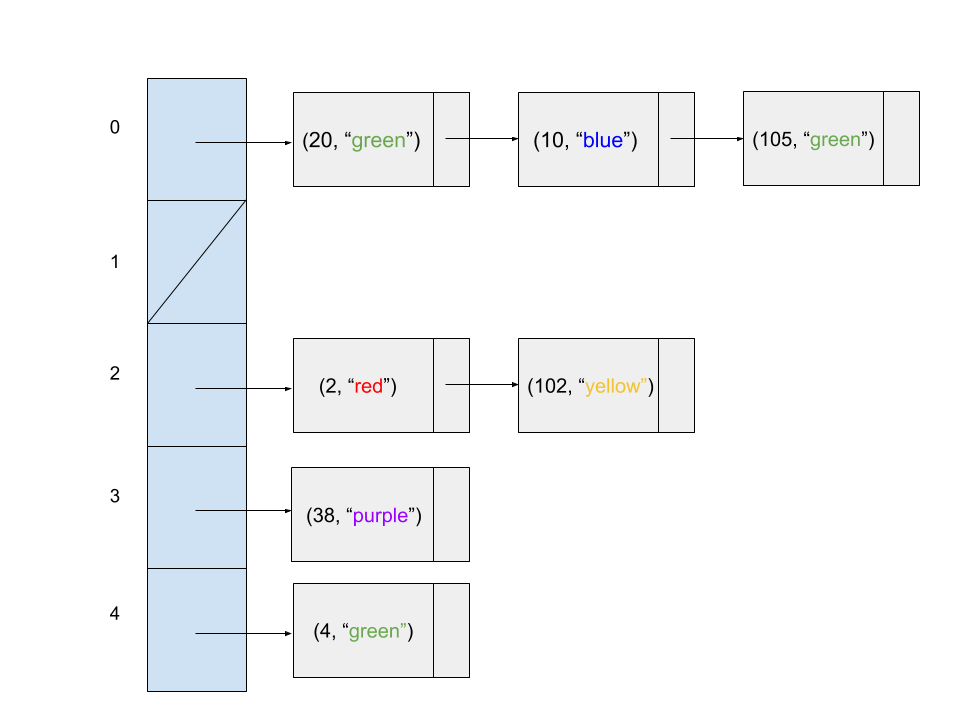•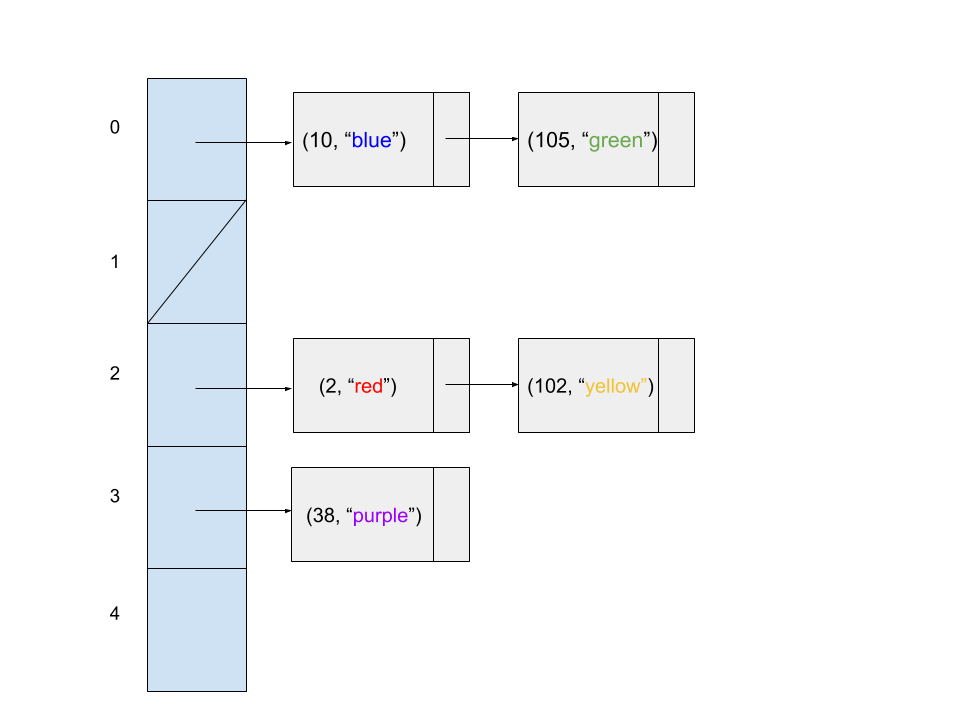•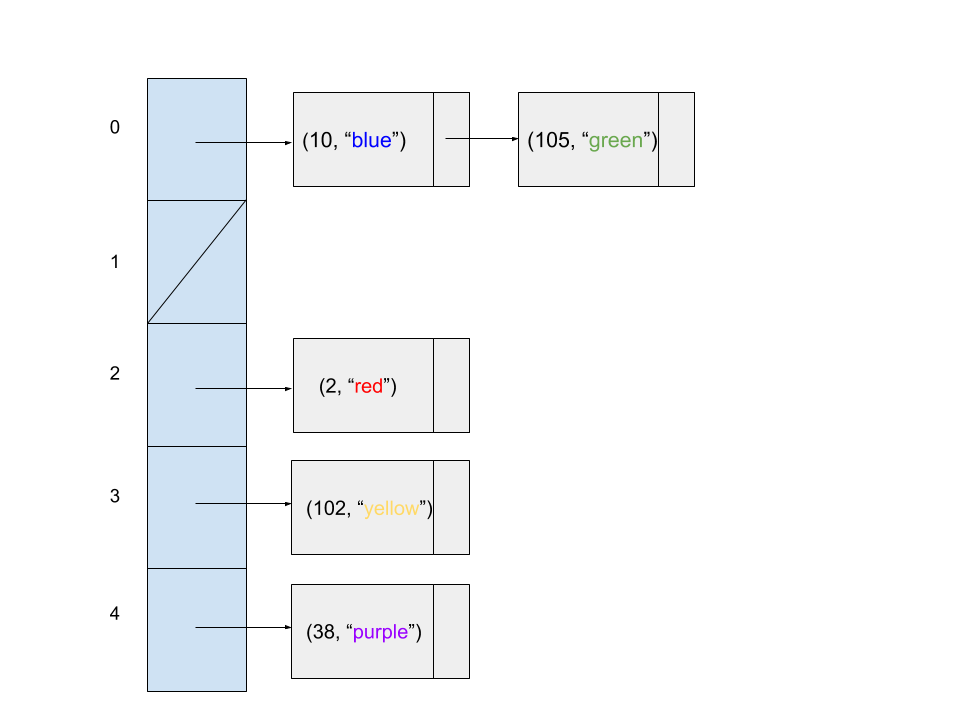### Question 2

Assuming the `HashMap` resizes its underlying array when the number of items equals the number of buckets by doubling the number of buckets (from 5 buckets to 10 buckets), which of the following diagrams might represent the state of the hash table at the end of the code snippet?

•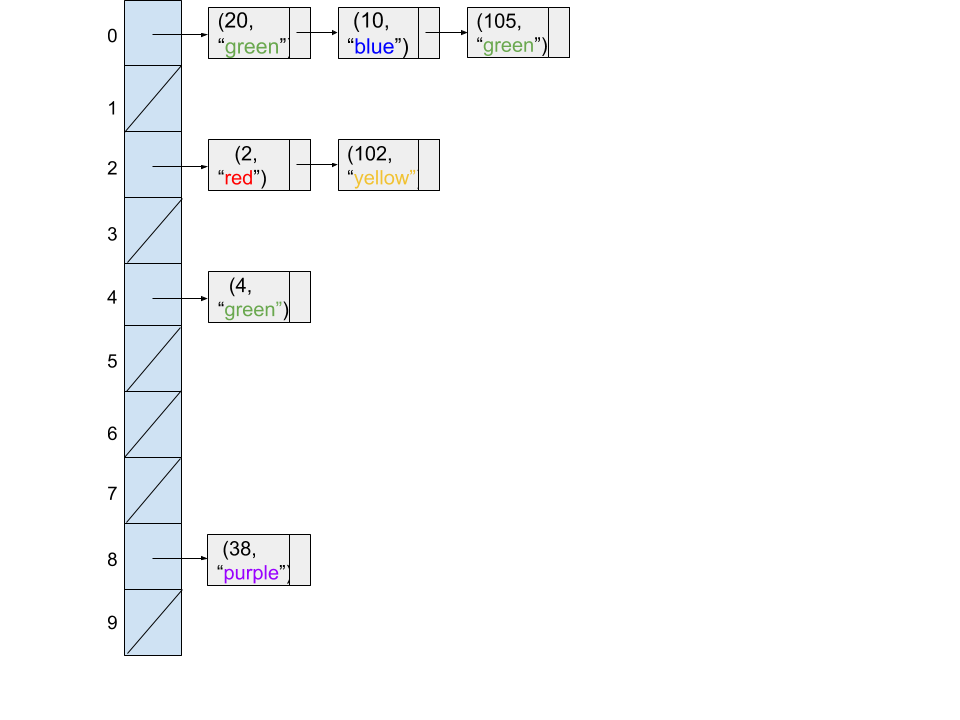•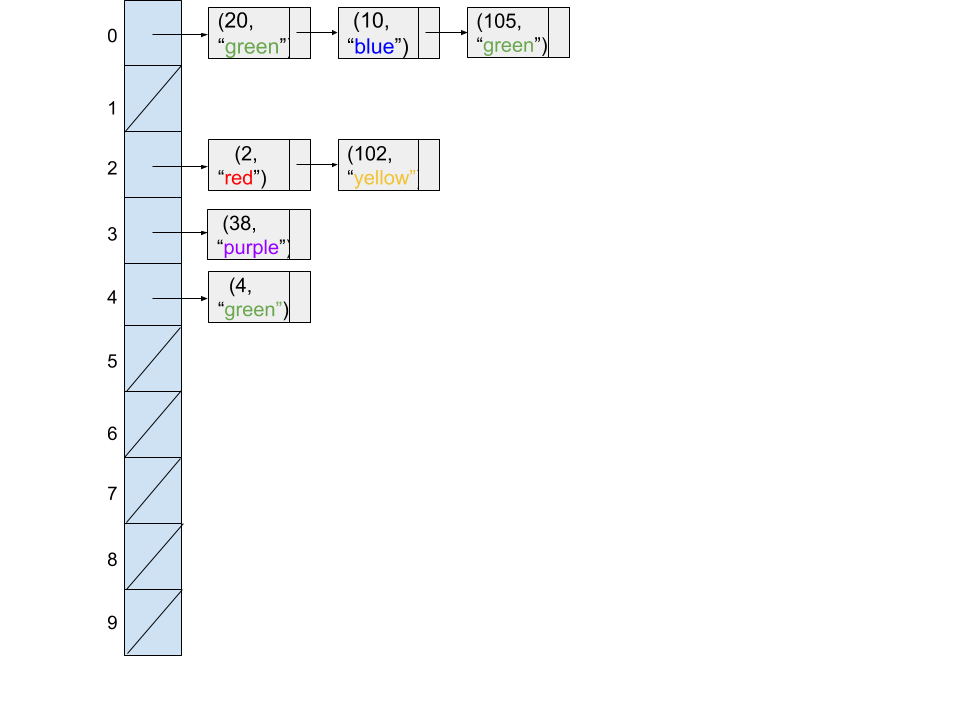•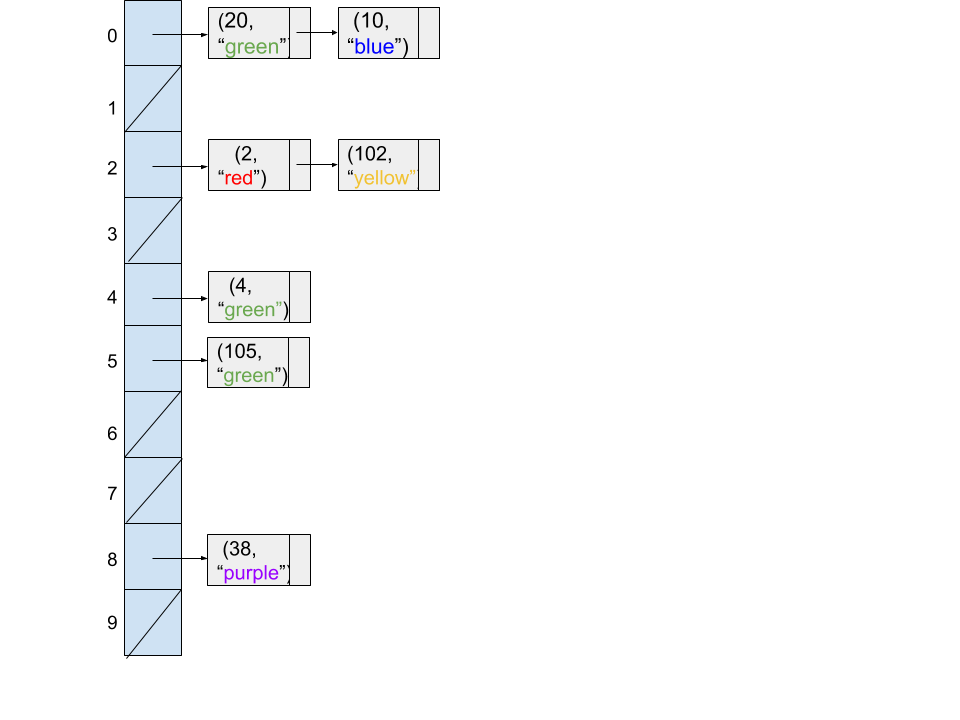## Hash functions

How does a Java `HashSet` or `HashMap` know how to compute each object’s hash code? Unlike search trees that relied on the `Comparable` interface, Java provides a default hash function implementation. All objects in Java provide a default implementation of the following two methods.

`boolean equals(Object o)`
Returns true if `o` is considered equal to this object. The default implementation checks that `this == o`.
`int hashCode()`
Returns the hash code for this object. The default implementation returns the memory address for this object.

The Java documentation for `Object.hashCode` describes the `hashCode` contract. When overriding the `equals` method, it is usual necessary to override the `hashCode` method so that it is consistent with `equals`.

### Question 1

True/false: Suppose we have a `Dog` class where each `Dog` has a `name` and a `weight`. What must the code output if we want the `Dog` class to work in a hash table?

``````Dog d1 = new Dog("Zeus", 5);
Dog d2 = new Dog("Zeus", 5);
// d1.equals(d2) evaluates to true!

System.out.println(d1.hashCode() == d2.hashCode());
``````
Explanation

If any two objects are equal, then they must also have the same exact hash code. Otherwise, if they do not have the same hash code, then they might not go to the same bucket in the hash table.

### Question 2

Suppose the `Dog` class overrides `equals` but not `hashCode`. What could happen if we run the following code?

``````Dog d1 = new Dog("Zeus", 5);
Dog d2 = new Dog("Zeus", 5);
// d1.equals(d2) evaluates to true!

Set<Dog> dogs = new HashSet<>();
System.out.println(dogs.contains(d2));
``````
• `d1` and `d2` will always have the same `hashCode`.
• `d1` and `d2` may have the same `hashCode`.
• `d1` and `d2` will have only a tiny chance of having the same `hashCode`.
• The print statement will always print false.
• The print statement will always print true.
• The print statement will sometimes print false and sometimes print true.
Explanation

`d1` and `d2` will not have the same `hashCode` since they are separate objects in memory. However, even though their hash codes will not directly collide, because the hash table computes the bucket index by taking the modulo of the hash code, it’s possible that they might just coincidentally happen to fall into the same bucket even though they have different hash codes.

### Question 3

True/false: Suppose the `Dog` class overrides `hashCode` but not `equals`. Will the following `hashCode` work in a hash table?

``````public int hashCode() {
return 17;
}
``````
Explanation

While this hash function technically works, all objects will go to the same bucket.

### Question 4

`ArrayList` provides valid and effective implementations for `equals` and `hashCode`. Why might we still caution storing `ArrayList` objects in a `HashSet`, i.e. `HashSet<ArrayList<String>>`?

• In a hash table, each bucket is a list of items, and you can’t store a list in a list.
• A list can be changed after its creation, so if one of the lists changes, it can get lost in the hash table.
• Lists can be potentially infinitely large but integer hash codes are finite, so collisions are very likely.

## LLRB tree buckets

We know that the worst-case runtimes for separate chaining hash table operations are closely related to the size of the largest bucket. In the hash table analysis, we compared hash tables without resizing (fixed number of buckets) versus hash tables with resizing.

Hash tables without resizing
The number of buckets is constant, so the size of the largest bucket is in Θ(N) with respect to N, the total size of the hash table.
Hash tables with resizing
The number of buckets grows linearly with respect to N. Assuming elements are evenly spread across the buckets, then the size of the largest bucket is in Θ(1).

In reality, Java `HashSet` and `HashMap` include a special optimization for avoiding worst-case runtime. When a linked list bucket grows contains more than 8 elements, the bucket will be converted into a left-leaning red-black tree. While this optimization does not affect the size of the largest bucket, it might improve the runtime.

For these problems, do not assume elements are evenly spread across the buckets.

### Question 1

Consider a hash table without resizing but using LLRB trees as the bucket data structure. Identify the worst-case runtime for inserting an element into this hash table with respect to N, the total size of the hash table.

• Θ(1)
• Θ(log N)
• Θ(N)
• Θ(Nlog N)
• Θ(N2)
Explanation

In the worst-case, all elements go to the same bucket. Thus, inserting into this hash table follows the same analysis as the worst-case for inserting into an LLRB tree.

### Question 2

Consider a hash table with resizing but using LLRB trees as the bucket data structure. Identify the worst-case runtime for inserting an element into this hash table with respect to N, the total size of the hash table.

• Θ(1)
• Θ(log N)
• Θ(N)
• Θ(Nlog N)
• Θ(N2)
Explanation

In the worst-case, all elements go to the same bucket and the insertion triggers a resize operation! Thus, inserting into this hash table follows the same analysis as the worst-case for inserting all N items into an LLRB tree.

### Question 2

Consider a hash table with resizing but using LLRB trees as the bucket data structure. Identify the worst-case runtime for inserting an element into this hash table with respect to N, the total size of the hash table.

• Θ(1)
• Θ(log N)
• Θ(N)
• Θ(Nlog N)
• Θ(N2)
Explanation

In the worst-case, all elements go to the same bucket and the insertion triggers a resize operation! Thus, inserting into this hash table follows the same analysis as the worst-case for inserting all N items into an LLRB tree.

### Question 3

A team member points out that this idea doesn’t actually help with the worst-case runtime for hash tables with resizing, so we shouldn’t use this idea. Do you agree with their assessment? Why might the Java developers implement this optimization in spite of the absolute worst-case runtime?